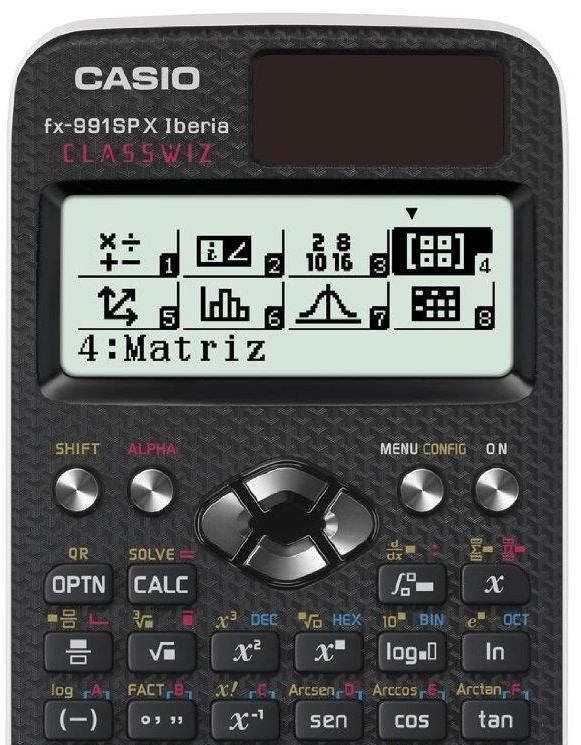Hexadecimal to Decimal Converter so that you can work with these two numerical systems so widely used in jobs and studies related to computer science.

After clicking on the calculate button, our calculator will provide you with the equivalent code in decimal. Finally, we would like to remind you that we also have available a decimal to hexadecimal converter.

## How to convert from hexadecimal to decimalChange from hexadecimal to decimal manually is very simple. You simply have to know that the hexadecimal system works in base 16. Once you have this clear, what you have to do is:

• Write the correspondence in decimal system of each symbol in hexadecimal. If you do not know what it is, you can consult this table
• Multiply each digit by its corresponding exponential in base 16. We will always start with exponent 0 for the digit that is more to the right and we will increase one unit as we advance towards the left. This you will understand it better seeing the solved exercise that you have in the following point.
• Add up all the multiplications we have done in the previous step.

In the following example you will see more clearly the operations that you must follow to pass the 3B code in hexadecimal to decimal format.

First of all find the correspondence in the decimal system of each symbol. The 3 in hexadecimal is 3 in decimal, however, the letter B in hexadecimal is equivalent to 11 in decimal.

Now we multiply their equivalents in decimal by their exponential in base 16 and add the results:

3B16 = 3×161+11×160 = 48+11 = 59

Let's see another solved exercise in which we are going to convert the symbol FAB to decimal. We return to look for the equivalences in the decimal system:

• F16 = 1510
• A16 = 1010
• B16 = 1110

Now we only have to multiply and add as we have seen in the previous example:

FAB16 = 15×162+ 10×161 + 11×160 = 3840 + 160 + 11 = 4011

If you have any doubts about which is the procedure to convert from hexadecimal to decimal write us a comment and we will help you as soon as possible.

## How to convert from hexadecimal to decimal in Excel

If you don't feel like doing the calculations for convert from hex to decimal and you do not have our calculator at hand, you can also use Excel to make the conversion.

You just have to open a new spreadsheet and set the following function in an empty cell:

=HEX2DEC()

Between the brackets you must write the number in quotation marks for Excel to do the conversion, otherwise it will return the argument #¡num, a sign that we have not put the number correctly. formula to convert from hexadecimal to decimal.

For example, if you want to pass the FF symbol in hexadecimal system, you have to put the formula as follows:

=HEX2DEC("FF")

## Hexadecimal to Decimal Equivalence Table

Below you will find a table with a list of all the symbols of the hexadecimal system and shows you the correspondence in the decimal system.. In addition, we have added some additional examples so that you can have a clearer idea of the equivalence between the two:

00
11
22
33
44
55
66
77
88
99
A10
B11
C12
D13
E14
F15
1016
2032
3048
4064
5080
6096
70112
80128
90144
A0160
B0176
C0192
D0208
E0224
F0240
100256
200512
300768
4001024

## Hexadecimal to decimal with calculatorIf none of the methods that we have seen above for change from hexadecimal to decimal convince you, we are going to propose you one more: use the scientific calculator. Many models for several years already offer the possibility of converting codes in different number systems and going from hexadecimal to decimal is possible.

To find out how this is done, we will use a Casio calculator as they are the most common in every home.

The steps to follow to convert from hexadecimal to decimal with calculator are:

1. Press the "Menu Config" or "Mode Setup" key, usually located in the upper right corner.
2. Select the "Base-N" option
3. Press the key with "HEX" at the top. In the calculator of the photo you have it in the penultimate row of buttons.
4. Type the hexadecimal number you want to convert to decimal. You can type the letters by pressing the keys on the last row of buttons on the calculator in the picture (at the top you will see an A, B, C, D, E or F).
5. When you have typed the number, press the = key.
6. Then press the key with a "DEC" on top of it.

If you have done the process correctly, the hexadecimal to decimal conversion correctly.

### 2 thoughts on “Hexadecimal a decimal”

1.Laura García Baidez

I have several doubts:
1° how to convert the octal number 156 to decimal and its explanation
2° how to convert the decimal number 195 to hexadecimal and its explanation
3° how to convert the number A7 to hexadecimal and its explanation

2.